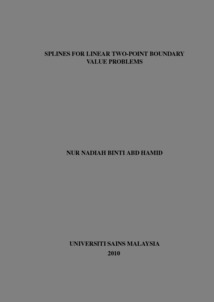# Splines For Linear Two-Point Boundary Value Problems

Hamid, Nur Nadiah Abd (2010) Splines For Linear Two-Point Boundary Value Problems. Masters thesis, Universiti Sains Malaysia.Preview
PDF
Download (216kB) | Preview

## Abstract

Linear two-point boundary value problems of order two are solved using cubic trigonometric B-spline, cubic Beta-spline and extended cubic B-spline interpolation methods. Cubic Beta-spline has two shape parameters, b1 and b2 while extended cubic B-spline has one, l . In this method, the parameters were varied and the corresponding approximations were compared to the exact solution to obtain the best values of b1, b2 and l . The methods were tested on four problems and the obtained approximated solutions were compared to that of cubic B-spline interpolation method. Trigonometric B-spline produced better approximation for problems with trigonometric form whereas Beta-spline and extended cubic B-spline produced more accurate approximation for some values of b1, b2 and l . All in all, extended cubic B-spline interpolation produced the most accurate solution out of the three splines. However, the method of finding l cannot be applied in the real world because there is no exact solution provided. That method was implemented in order to test whether values of l that produce better approximation do exist. Thus, an approach of finding optimized l is developed and Newton’s method was applied to it. This approach was found to approximate the solution much better than cubic B-spline interpolation method.

Item Type: Thesis (Masters) Q Science > QA Mathematics > QA101-145 Elementary Mathematics, Arithmetic Pusat Pengajian Sains Matematik (School of Mathematical Sciences) > Thesis HJ Hazwani Jamaluddin 04 Sep 2018 07:21 12 Apr 2019 05:26 http://eprints.usm.my/id/eprint/41694

### Actions (login required)View Item